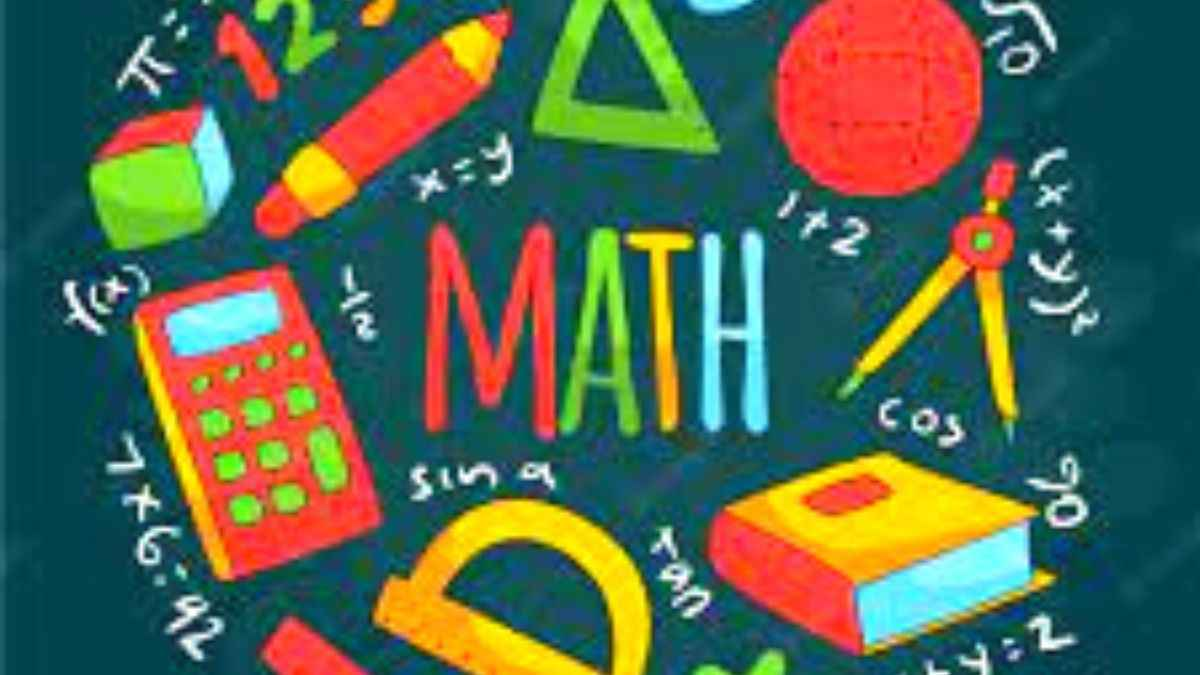# Math riddles with answers: These math riddles might give you a reality-check! Try these math riddles.

Everybody is a gangster unless challenging math riddles come. Can you solve these math riddles?Math riddles are a fun way to check how smart one is.

Can you solve these exciting math riddles?

Math Riddle 1:

What is so unique about the number 854,917,632?

Math Riddle 2:

Who am I? I am a three-digit number. My ones digit is six less than my tens digit. My tens digit is eight more than my hundreds digit. Can you guess the number?

Math Riddle 3:

Arrange four 9s in such a way that it becomes equal to 100.

Here are the answers you are seeking!

Math Riddle 1:

What is so unique about the number 854,917,632?

The numbers are arranged from one to nine in alphabetical order.

Math Riddle 2:

Who am I? I am a three-digit number. My ones digit is six less than my tens digit. My tens digit is eight more than my hundreds digit. Can you guess the number?

193

Math Riddle 3:

Arrange four 9s in such a way that it becomes equal to 100.